# 使用长短期记忆网络对 ECG 信号进行分类

### 简介

ECG 记录一段时间内人体心脏的电活动。医生使用 ECG 检测患者的心跳是正常还是不规则。

### 加载并检查数据

```if ~isfile('PhysionetData.mat') ReadPhysionetData end load PhysionetData```

`Signals(1:5)`
```ans=5×1 cell array {1×9000 double} {1×9000 double} {1×18000 double} {1×9000 double} {1×18000 double} ```
`Labels(1:5)`
```ans = 5×1 categorical N N N A A ```

`summary(Labels)`
``` A 738 N 5050 ```

```L = cellfun(@length,Signals); h = histogram(L); xticks(0:3000:18000); xticklabels(0:3000:18000); title('Signal Lengths') xlabel('Length') ylabel('Count')```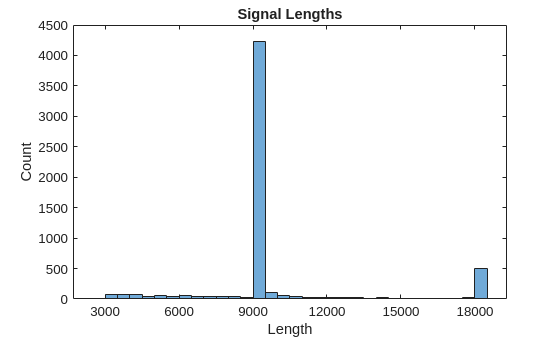```normal = Signals{1}; aFib = Signals{4}; subplot(2,1,1) plot(normal) title('Normal Rhythm') xlim([4000,5200]) ylabel('Amplitude (mV)') text(4330,150,'P','HorizontalAlignment','center') text(4370,850,'QRS','HorizontalAlignment','center') subplot(2,1,2) plot(aFib) title('Atrial Fibrillation') xlim([4000,5200]) xlabel('Samples') ylabel('Amplitude (mV)')```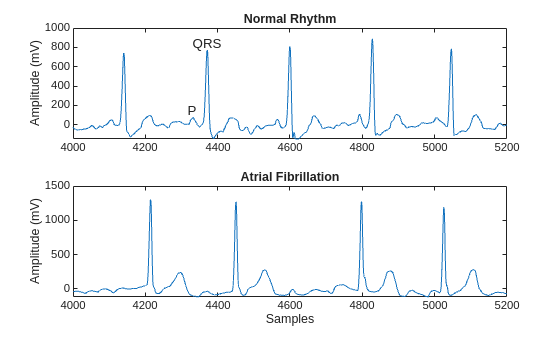### 准备用于训练的数据

`[Signals,Labels] = segmentSignals(Signals,Labels);`

`Signals(1:5)`
```ans=5×1 cell array {1×9000 double} {1×9000 double} {1×9000 double} {1×9000 double} {1×9000 double} ```

### 使用原始信号数据训练分类器

`summary(Labels)`
``` A 718 N 4937 ```

```afibX = Signals(Labels=='A'); afibY = Labels(Labels=='A'); normalX = Signals(Labels=='N'); normalY = Labels(Labels=='N');```

```[trainIndA,~,testIndA] = dividerand(718,0.9,0.0,0.1); [trainIndN,~,testIndN] = dividerand(4937,0.9,0.0,0.1); XTrainA = afibX(trainIndA); YTrainA = afibY(trainIndA); XTrainN = normalX(trainIndN); YTrainN = normalY(trainIndN); XTestA = afibX(testIndA); YTestA = afibY(testIndA); XTestN = normalX(testIndN); YTestN = normalY(testIndN);```

```XTrain = [repmat(XTrainA(1:634),7,1); XTrainN(1:4438)]; YTrain = [repmat(YTrainA(1:634),7,1); YTrainN(1:4438)]; XTest = [repmat(XTestA(1:70),7,1); XTestN(1:490)]; YTest = [repmat(YTestA(1:70),7,1); YTestN(1:490);];```

`summary(YTrain)`
``` A 4438 N 4438 ```
`summary(YTest)`
``` A 490 N 490 ```

### 定义 LSTM 网络架构

LSTM 网络可以学习序列数据的时间步之间的长期相关性。此示例使用双向 LSTM 层 `bilstmLayer`，因为它前向和后向检测序列。

```layers = [ ... sequenceInputLayer(1) bilstmLayer(100,'OutputMode','last') fullyConnectedLayer(2) softmaxLayer classificationLayer ]```
```layers = 5x1 Layer array with layers: 1 '' Sequence Input Sequence input with 1 dimensions 2 '' BiLSTM BiLSTM with 100 hidden units 3 '' Fully Connected 2 fully connected layer 4 '' Softmax softmax 5 '' Classification Output crossentropyex ```

```options = trainingOptions('adam', ... 'MaxEpochs',10, ... 'MiniBatchSize', 150, ... 'InitialLearnRate', 0.01, ... 'SequenceLength', 1000, ... 'GradientThreshold', 1, ... 'ExecutionEnvironment',"auto",... 'plots','training-progress', ... 'Verbose',false);```

### 训练 LSTM 网络

`net = trainNetwork(XTrain,YTrain,layers,options);`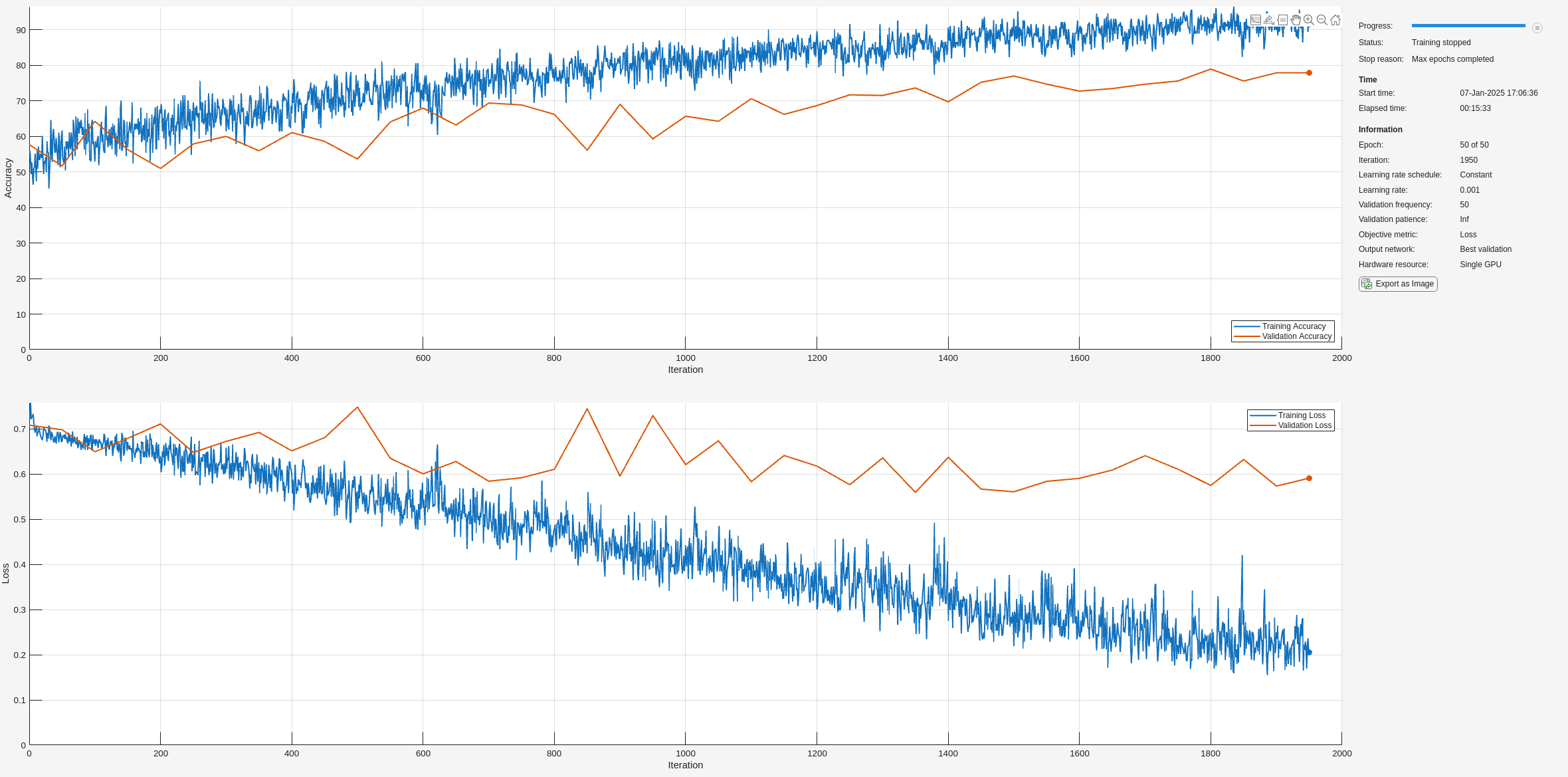### 可视化训练和测试准确度

`trainPred = classify(net,XTrain,'SequenceLength',1000);`

`LSTMAccuracy = sum(trainPred == YTrain)/numel(YTrain)*100`
```LSTMAccuracy = 61.7283 ```
```figure confusionchart(YTrain,trainPred,'ColumnSummary','column-normalized',... 'RowSummary','row-normalized','Title','Confusion Chart for LSTM');```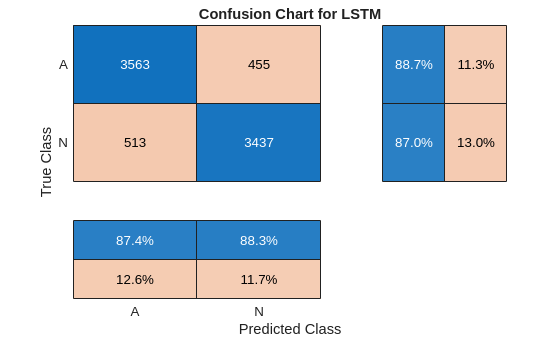`testPred = classify(net,XTest,'SequenceLength',1000);`

`LSTMAccuracy = sum(testPred == YTest)/numel(YTest)*100`
```LSTMAccuracy = 66.2245 ```
```figure confusionchart(YTest,testPred,'ColumnSummary','column-normalized',... 'RowSummary','row-normalized','Title','Confusion Chart for LSTM');```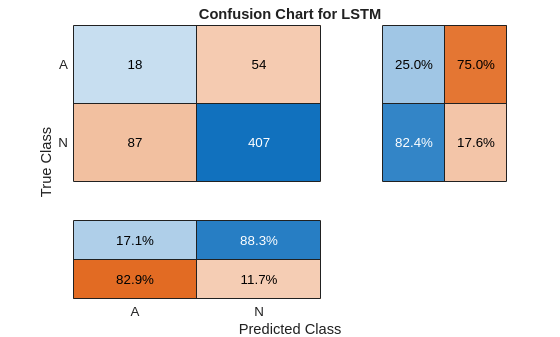### 通过特征提取提高性能

```fs = 300; figure subplot(2,1,1); pspectrum(normal,fs,'spectrogram','TimeResolution',0.5) title('Normal Signal') subplot(2,1,2); pspectrum(aFib,fs,'spectrogram','TimeResolution',0.5) title('AFib Signal')```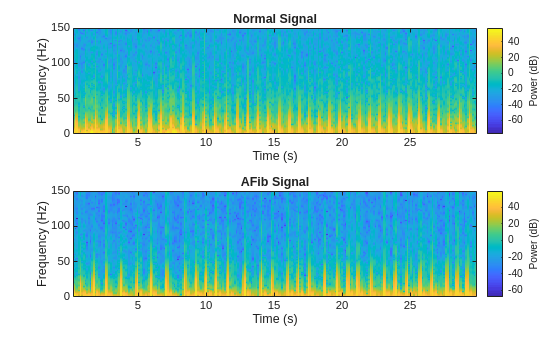• 瞬时频率 (`instfreq`)

• 谱熵 (`pentropy`)

`instfreq` 函数估计信号的时变频率，作为功率谱图的第一个矩。该函数使用时间窗上的短时傅里叶变换计算频谱图。在本示例中，该函数使用 255 个时间窗。该函数的时间输出对应于时间窗的中心。

```[instFreqA,tA] = instfreq(aFib,fs); [instFreqN,tN] = instfreq(normal,fs); figure subplot(2,1,1); plot(tN,instFreqN) title('Normal Signal') xlabel('Time (s)') ylabel('Instantaneous Frequency') subplot(2,1,2); plot(tA,instFreqA) title('AFib Signal') xlabel('Time (s)') ylabel('Instantaneous Frequency')```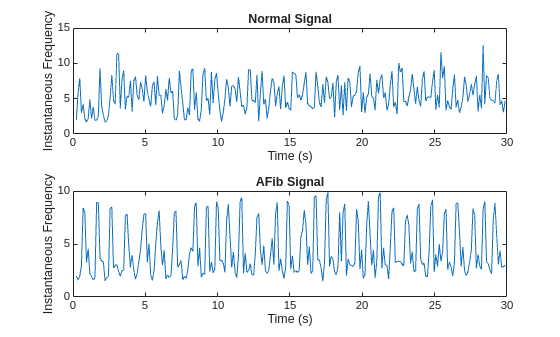```instfreqTrain = cellfun(@(x)instfreq(x,fs)',XTrain,'UniformOutput',false); instfreqTest = cellfun(@(x)instfreq(x,fs)',XTest,'UniformOutput',false);```

```[pentropyA,tA2] = pentropy(aFib,fs); [pentropyN,tN2] = pentropy(normal,fs); figure subplot(2,1,1) plot(tN2,pentropyN) title('Normal Signal') ylabel('Spectral Entropy') subplot(2,1,2) plot(tA2,pentropyA) title('AFib Signal') xlabel('Time (s)') ylabel('Spectral Entropy')```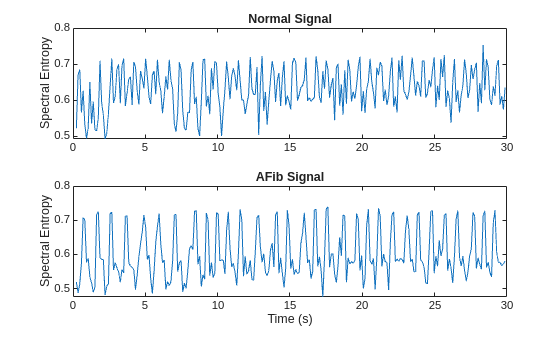```pentropyTrain = cellfun(@(x)pentropy(x,fs)',XTrain,'UniformOutput',false); pentropyTest = cellfun(@(x)pentropy(x,fs)',XTest,'UniformOutput',false);```

```XTrain2 = cellfun(@(x,y)[x;y],instfreqTrain,pentropyTrain,'UniformOutput',false); XTest2 = cellfun(@(x,y)[x;y],instfreqTest,pentropyTest,'UniformOutput',false);```

`XTrain2(1:5)`
```ans=5×1 cell array {2×255 double} {2×255 double} {2×255 double} {2×255 double} {2×255 double} ```

### 标准化数据

`mean(instFreqN)`
```ans = 5.5615 ```
`mean(pentropyN)`
```ans = 0.6326 ```

```XV = [XTrain2{:}]; mu = mean(XV,2); sg = std(XV,[],2); XTrainSD = XTrain2; XTrainSD = cellfun(@(x)(x-mu)./sg,XTrainSD,'UniformOutput',false); XTestSD = XTest2; XTestSD = cellfun(@(x)(x-mu)./sg,XTestSD,'UniformOutput',false);```

```instFreqNSD = XTrainSD{1}(1,:); pentropyNSD = XTrainSD{1}(2,:); mean(instFreqNSD)```
```ans = -0.3211 ```
`mean(pentropyNSD)`
```ans = -0.2416 ```

### 修改 LSTM 网络架构

```layers = [ ... sequenceInputLayer(2) bilstmLayer(100,'OutputMode','last') fullyConnectedLayer(2) softmaxLayer classificationLayer ]```
```layers = 5x1 Layer array with layers: 1 '' Sequence Input Sequence input with 2 dimensions 2 '' BiLSTM BiLSTM with 100 hidden units 3 '' Fully Connected 2 fully connected layer 4 '' Softmax softmax 5 '' Classification Output crossentropyex ```

```options = trainingOptions('adam', ... 'MaxEpochs',30, ... 'MiniBatchSize', 150, ... 'InitialLearnRate', 0.01, ... 'GradientThreshold', 1, ... 'ExecutionEnvironment',"auto",... 'plots','training-progress', ... 'Verbose',false);```

### 用时频特征训练 LSTM 网络

`net2 = trainNetwork(XTrainSD,YTrain,layers,options);`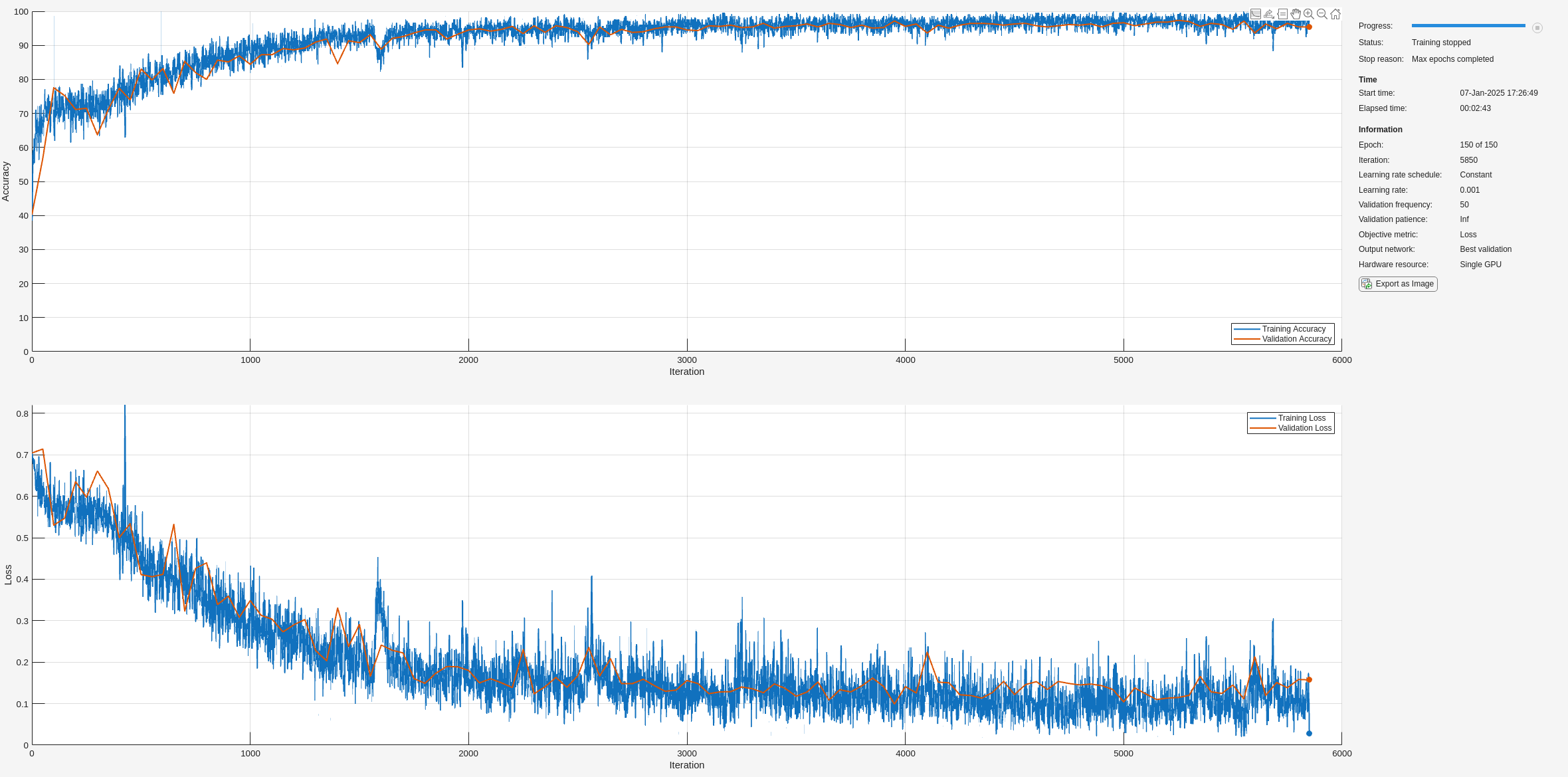### 可视化训练和测试准确度

```trainPred2 = classify(net2,XTrainSD); LSTMAccuracy = sum(trainPred2 == YTrain)/numel(YTrain)*100```
```LSTMAccuracy = 83.5962 ```
```figure confusionchart(YTrain,trainPred2,'ColumnSummary','column-normalized',... 'RowSummary','row-normalized','Title','Confusion Chart for LSTM');```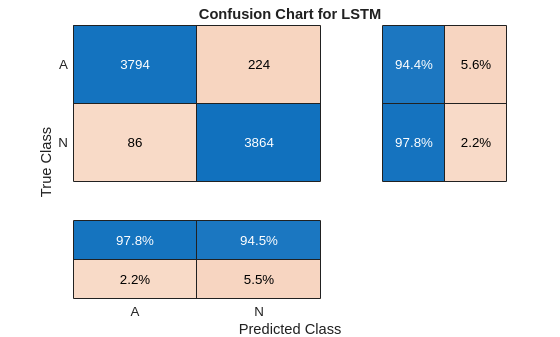```testPred2 = classify(net2,XTestSD); LSTMAccuracy = sum(testPred2 == YTest)/numel(YTest)*100```
```LSTMAccuracy = 80.1020 ```
```figure confusionchart(YTest,testPred2,'ColumnSummary','column-normalized',... 'RowSummary','row-normalized','Title','Confusion Chart for LSTM');```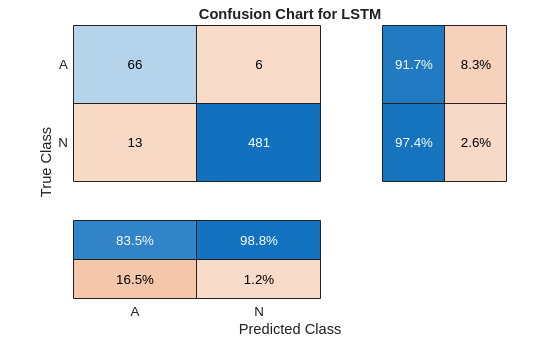### 参考资料

 AF Classification from a Short Single Lead ECG Recording: the PhysioNet/Computing in Cardiology Challenge, 2017. https://physionet.org/challenge/2017/

 Clifford, Gari, Chengyu Liu, Benjamin Moody, Li-wei H. Lehman, Ikaro Silva, Qiao Li, Alistair Johnson, and Roger G. Mark."AF Classification from a Short Single Lead ECG Recording:The PhysioNet Computing in Cardiology Challenge 2017."Computing in Cardiology (Rennes:IEEE).Vol. 44, 2017, pp. 1–4.

 Goldberger, A. L., L. A. N. Amaral, L. Glass, J. M. Hausdorff, P. Ch.Ivanov, R. G. Mark, J. E. Mietus, G. B. Moody, C.-K. Peng, and H. E. Stanley."PhysioBank, PhysioToolkit, and PhysioNet:Components of a New Research Resource for Complex Physiologic Signals".Circulation.Vol. 101, No. 23, 13 June 2000, pp. e215–e220. http://circ.ahajournals.org/content/101/23/e215.full

 Pons, Jordi, Thomas Lidy, and Xavier Serra."Experimenting with Musically Motivated Convolutional Neural Networks".14th International Workshop on Content-Based Multimedia Indexing (CBMI).June 2016.

 Wang, D."Deep learning reinvents the hearing aid," IEEE Spectrum, Vol. 54, No. 3, March 2017, pp. 32–37. doi:10.1109/MSPEC.2017.7864754.

 Brownlee, Jason.How to Scale Data for Long Short-Term Memory Networks in Python.7 July 2017. https://machinelearningmastery.com/how-to-scale-data-for-long-short-term-memory-networks-in-python/.